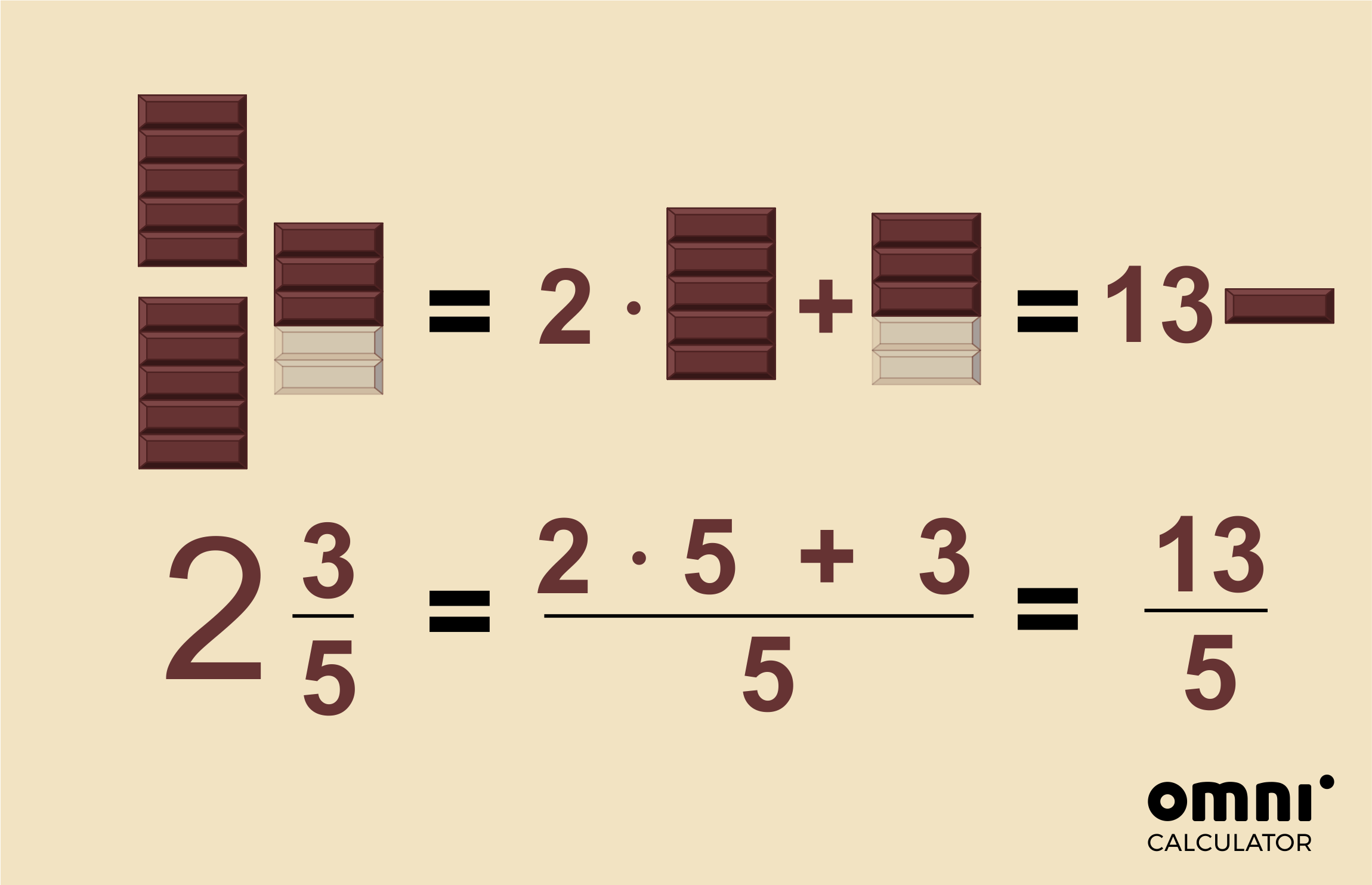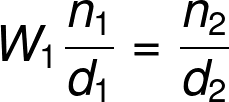# Mixed Number to Improper Fraction Calculator

Created by Hanna Pamuła, PhD candidate
Reviewed by Dominik Czernia, PhD candidate and Jack Bowater
Last updated: Jul 27, 2020

Welcome to the mixed number to improper fraction calculator - a tool that can convert fractions in the blink of an eye 😉 If you're wondering how to change a mixed number into an improper fraction, you're in the right place - below, you'll find a short explanation on how to do it by hand. Also, the calculator itself has a step-by-step guide👣, so don't worry too much about your homework - we'll give you a helping hand when converting mixed numbers to improper fractions!

If you're on the hunt for the opposite conversion, make sure to check our improper fraction to mixed number calculator.

## How to change a mixed number into an improper fraction?

There are only three steps required when converting mixed numbers to improper fractions. As an example, let's take 2 3/5 and convert it to an improper fraction:

Step 1

Multiply the whole number by the denominator of the fractional part:

2 * 5 = 10

Step 2

10 + 3 = 13

Step 3

The number you just calculated is your new numerator - put it on top of your original denominator:

13/5

In this case, the improper fraction is already in its simplest form.

However, it may happen that you need to reduce the fraction. For example, if the result is 28/10, you need to find the greatest common factor (GCF), and divide both numbers by it:

GCF(28,10) = 2

28/10 = 14/5

Are you still not sure how to change a mixed number into an improper fraction? Let's take a look at a visual example...

## Converting mixed numbers to improper fractions - visually 👀

To make it easier to understand and remember, we'll illustrate the conversion of 2 3/5 again. Let's immerse ourselves into the world of chocolate:In the beginning, you need to find out how many rows are in the whole chocolate bars:

2 whole chocolate bars, each bar has 5 rows:

2 * 5 = 10

Next, add the 3 rows from the incomplete bar:

10 + 3 = 13

Finally, write the number as an improper fraction:

13/5

Why do you need to divide by 5 (or, in other words, multiply by 1/5)? Well, that's because each row is a 1/5 of the whole chocolate bar!

So why is converting mixed numbers to improper fractions so useful and important? Imagine you have the chocolate bars from the picture, exactly 2 3/5 full chocolate bars. You'd like to share your grub with your classmates 🧒🧒🏻🧒🏽. If each person gets one row, how many people can you share it out between?

We're sure you guessed that - 13 people! Exactly 13 kids can have one row of chocolate.

## Example: convert 3 ¹/₄ to an improper fraction with the mixed number to improper fraction calculator

Using this mixed number to improper fraction calculator is a piece of cake 🍰, but we want to be sure it's crystal clear for you. We'll show you how the tool works on one example: let's say that you'd like to turn 3 1/4 into its improper fraction form:

1. Input the whole part of the mixed number. In our case, the whole part is equal to 3.
2. Enter the fractional part. 1 as the numerator and 4 as the denominator.
3. Sit back and relax😎 - the tool displays the result for you, immediately!
Mixed
number
Improper
fraction
3 1/4 = 13/4
1. If some part of the conversion is still puzzling you, make sure to check out the step-by-step solution that we display directly below the mixed number to improper fraction calculator.
Hanna Pamuła, PhD candidateWhole number (W₁)
Numerator (n₁)
Denominator (d₁)
Step by step solution?
No
People also viewed…

### Average percentage

The average percentage calculator can take up to ten percentage values with possibly different sample sizes and return the average percentage of the entire dataset.

### Circle skirt

Circle skirt calculator makes sewing circle skirts a breeze.

### Circumference

Use this free circumference calculator to find the area, circumference and diameter of a circle.

### Gram-Schmidt

The Gram-Schmidt calculator is a quick and easy-to-use tool that uses the orthogonalization process to find the orthonormal basis of the space spanned by your vectors.